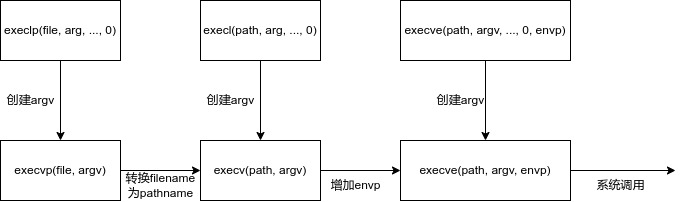# Unix网络编程（2）：基本TCP套接字编程

## 1. 基本TCP套接字编程

### 1.1 socket函数

为了执行网络I/O，进程必须做的第一件事就是调用 $socket$ 函数。

#include <sys/socket.h>

// 成功返回套接字描述符，失败返回-1
int socket(int family, int type. int protocol);


$family$ 为协议族，由于历史问题，一些实现中还存在以 $PF$ 开头的协议族，但基本等价；$type$ 指明套接字类型；$protocol$ 为某个协议类型常值。

$family$ 说明
$AF_-INET$ IPv4协议
$AF_-INET6$ IPv6协议
$AF_-LOCAL$ Unix域协议
$AF_-ROUTE$ 路由套接字
$AF_-KEY$ 密钥套接字

$type$ 说明
$SOCK_-STREAM$ 字节流套接字
$SOCK_-DGRAM$ 数据报套接字
$SOCK_-SEQPACKET$ 有序分组套接字
$SOCK_-RAW$ 原始套接字

$protocol$ 说明
$IPPROTO_-TCP$ TCP传输协议
$IPPROTO_-UDP$ UDP传输协议
$IPPROTO_-SCTP$ SCTP传输协议

$SOCK_-STREAM$ TCPSCTP TCPSCTP 有效
$SOCK_-DGRAM$ UDP UDP 有效
$SOCK_-SEQPACKET$ SCTP SCTP 有效
$SOCK_-RAW$ IPv4 IPv6 有效 有效

### 1.2 connect函数

TCP客户使用 $connect$ 函数来与TCP服务器建立连接。

#include <sys/socket.h>

// 成功返回0，否则返回-1


客户在调用 $connect$ 前不必非得调用 $bind$ 函数，因为如果需要的话，内核会确定源IP地址，并选择一个临时端口作为源端口。如果是TCP套接字，调用 $connect$ 将会触发TCP三次握手。如果客户端没有收到SYN的确认，会设置 $ETIMEDOUT$ ；如果SYN响应为RST，会设置 $ECONNREFUSED$ ；如果SYN数据包引发了 “$destination$ $unreachable$” ( 目的地不可达 ) 的ICMP错误，那么客户内核会保存信息并隔一定时间后重试，如果还是失败，则设置 $EHOSTUNREACH$ 或者 $ENETUNREACH$ 。

### 1.3 bind函数

$bind$ 函数将一个本地协议地址赋予一个套接字。

#include <sys/socket.h>

// 成功返回0，否则返回-1


如果一个TCP客户或服务器未曾调用 $bind$ ，那么当调用 $connect$ 或 $listen$ 时，内核会选择一个临时端口。但是对于TCP服务器，让内核选择端口是极为罕见的，这个规则的例外是RPC服务器。$bind$ 可以指定IP地址和端口中的一个，也可以两个都不指定。

IP地址 端口 结果

对于IPv4来说，通配地址通常由常数 $INADDR_-ANY$ 指定，一般为 $0$ 。而对于IPv6，由于其地址存放在结构中，所以系统会预先分配 $in6addr_-any$ 变量并初始化为常值 $IN6ADDR_-ANY_-INIT$ 。如果让内核选择端口号，那么 $bind$ 并不会返回对应值，需要调用 $getsockname$ 返回协议地址。
$bind$ 函数的一个常见错误是 $EADDRINUSE$ ，表示地址已被占用。

### 1.4 listen函数

$listen$ 函数仅由TCP服务器调用，

#include <sys/socket.h>

// 成功返回0，否则返回-1
int listen(int sockfd, int backlog);


当 $socket$ 函数创建一个套接字时，它被假设成一个主动套接字，即负责 $connect$ 的套接字，而 $listen$ 可以把它转换成一个被动套接字，即把TCP状态从 $CLOSED$ 转为 $LISTEN$ 。内核为任何一个给定的监听套接字维护两个队列：未完成队列 ( $incomplete$ $connection$ $queue$ ) 和已完成队列 ( $completed$ $connection$ $queue$ )，分别存储着未完成三次握手的套接字和已完成三次握手的套接字。$backlog$ 参数是一个因子，与队列长度有关。

### 1.5 accept函数

$accept$ 函数由TCP服务器调用，用于从已连接队列队头取出已完成的连接，如果队列为空，则默认会阻塞。

#include <sys/socket.h>

// 成功返回描述符，否则返回-1


如果 $accept$ 成功，其返回值是一个由内核自动生成的全新描述符，代表与所返回客户的TCP连接。我们称 $accept$ 的第一个参数为监听套接字描述符，返回值为已连接套接字描述符，在服务器生命周期内一直存在。如果对客户协议地址和长度不感兴趣，可以设为 $NULL$ 。

### 1.6 fork和exec函数

$fork$ 是Unix系统提供的函数，是Unix派生新进程的唯一方法。

#include <unistd.h>

// 子进程中返回值为0，父进程中为子进程PID，出错返回-1
pid_t fork(void);


任何子进程只有一个父进程，子进程可以通过 $getppid$ 函数获取父进程PID。父进程可以有多个子进程，如果父进程想跟踪子进程，就必须记录 $fork$ 函数的返回值。$fork$ 有两个典型用法：

1. 一个进程创建自身的副本，这样每个副本都可以在另一个副本执行其他任务的同时处理各自的操作，这也是网络服务器的典型用法；
2. 一个进程想要执行另一个程序，首先调用 $fork$ 创建一个自身的副本，然后副本调用 $exec$ 把自身替换成新的程序，这是诸如shell之类程序的典型用法。

存放在硬盘上的可执行程序能够被Unix执行的唯一方法是：由一个现有进程调用 $6$ 个 $exec$ 函数中的某一个，$exec$ 会把当前进程映像替换成新的程序文件，而且该新程序通常从 $main$ 函数开始执行。我们称调用 $exec$ 的进程为调用进程 ( $calling$ $process$ )，称新执行的程序为新程序 ( $new$ $program$ )。

#include <unistd.h>

int execl(const char *pathname, const char *arg0, ...);

int execv(const char *pathname, char *const *argv[]);

int execle(const char *pathname, const char *arg0, ...);

int execve(const char *pathname, char *const argv[], char *const envp[]);

int execlp(const char *filename, const char *arg0, ...);

int execvp(const char *filename, char *const argv[]);


这 $6$ 个函数的区别在于：待执行的程序文件是由文件名还是由路径名指定、新程序参数是一一列出还是由一个指针数组引用、是把调用进程的环境传递给新程序还是指定新环境。这些函数只有在出错时才会返回到调用者，否则控制会被传递给新程序的起始点，通常就是 $main$ 函数。### 1.7 并发服务器

Unix中编写并发服务器程序最简单的办法就是 $fork$ 一个子进程来服务每个客户。

pid_t pid;
int listenfd, connfd;

listenfd = Socket(...);
Bind(listenfd, ...);
Listen(listenfd, LISTENQ);

for (;;) {
connfd = Accept(listenfd, ...);
if ((pid = Fork()) == 0) {
Close(listenfd);
dosomething(connfd);
Close(connfd);
exit(0);
}
Close(connfd);
}


当一个连接建立时，$accept$ 返回，服务器调用 $fork$ ，然后子进程服务客户，父进程则关闭已连接套接字，然后等待另一个连接。由于进程终止前会关闭所有打开的描述符，所以在 $if$ 块内的 $exit$ 函数前的关闭是非必要的。父进程调用 $close$ 后子进程的连接并不会断开，因为每个套接字都有一个引用计数，维护在文件表中，调用 $close$ 会让引用计数减一。

### 1.8 close函数

通常的Unix $close$ 函数也用于关闭套接字，终止TCP连接。

#include <unistd.h>

// 成功返回0，否则返回-1
int close(int sockfd);


$close$ 一个TCP套接字的默认行为是把该套接字标记成关闭，然后立即返回到调用进程，之后该套接字描述符不能再用于 $read$ 和 $write$　。然而TCP仍然会尝试发送队列中的消息，发送完毕后才会正式关闭连接。如果套接字描述符的引用计数大于 $1$ ，那么 $close$ 不会引发TCP连接的关闭，而是会让引用计数减一。如果确实想在某个TCP连接上发送FIN，可以使用 $shutdown$ 函数。

## 9. getsockname和getpeername函数

#include <sys/socket.h>



这两个函数分别返回与某个套接字关联的本地协议地址或者外地协议地址。用途如下：

• 在没有 $bind$ 的TCP客户上在 $connect$ 成功返回后，通过 $getsockname$ 返回内核赋予的本地IP地址和端口号；
• 在以端口号 $0$ 调用 $bind$ 后，通过 $getsockname$ 返回内核赋予的本地端口号；
• $getsockname$ 可以获取某个套接字的地址族；
• 在以通配符调用 $bind$ 后，与某个客户的连接一旦建立，通过 $getsockname$ 返回内核赋予的本地IP地址。注意这时的套接字描述符参数必须是已连接套接字描述符；
• 服务器由调用过 $accept$ 的进程调用 $exec$ 后，能够确定客户身份的唯一方式便是调用 $getpeername$ 。

## 2. POSIX信号处理

### 2.1 信号语义

信号 ( $signal$ ) 就是告知某个进程发生了某个事件的通知，有时也被称为软件中断 ( $software$ $interrupt$ )。信号通常是异步发生的，也就是说进程预先不知道信号的准确发生时刻。信号可以：

• 由一个进程发给另一个进程 ( 或自身 )；
• 由内核发给某个进程。

每个信号都有一个与之关联的处置 ( $disposition$ )，也称为行为 ( $action$ )。一个信号的处置可以有三个选择：

1. 提供一个函数，只要有特定信号发生就被调用，我们称之为 信号处理函数 ( $signal$ $handler$ )，这种行为称为捕获 ( $catching$ ) 信号。有两个信号不能被捕获，分别是 $SIGKILL$ 和 $SIGSTOP$ 。信号处理函数由信号值这单一整型参数调用，没有返回值；
2. 把某个信号的处置设为 $SIG_-IGN$ 来忽略它，$SIGKILL$ 和 $SIGSTOP$ 不能被忽略；
3. 把某个信号的处置设定为 $SIG_-DFL$ 来启用它的默认处置，通常是终止进程，其中某些信号还会在当前工作目录产生一个进程的核心映像/内存影像 ( $core$ $image$ )，另有个别信号的默认处置是忽略，比如 $SIGCHILD$ 和 $SIGURG$ 。

建立信号处置的POSIX方法就是调用 $sigaction$ 函数，但是有些复杂，因为其第一个参数是一个结构。简单些的方法就是调用 $signal$ 函数，第一个参数是信号名，第二个参数或为函数指针，或为常值 $SIG_-IGN$ 和 $SIG_-DFL$ 。然而 $signal$ 是早于 POSIX出现的函数，因此不同的实现会有不同的语义，一种方法是定义自己的 $signal$ 函数。

typedef void Sigfunc(int);

Sigfunc *signal(int signo, Sigfunc *func) {
struct sigaction act, oact;
act.sa_handler = func;
act.sa_flags = 0;
if (signo == SIGALRM) {
#ifdef SA_INTERRUPT
act.sa_flags |= SA_INTERRUPT;
#endif
} else {
#ifdef SA_RESTART
act.sa_flags |= SA_RESTART;
#endif
}
if (sigaction(signo, &act, &oact) < 0)
return SIG_ERR;
return oact.sa_handler;
}


我们把符合POSIX的系统上的信号处理总结为以下几点：

• 一旦安装了信号处理函数，便一直存在；
• 在一个信号处理函数运行期间，正被递交的信号是阻塞的。而且，安装函数时在传递给 $sigaction$ 函数的 $sa_-mask$ 信号集中指定的额外信号也被阻塞；
• 如果一个信号在被阻塞期间产生，那么该信号在被解阻塞后只递交一次，也就是默认不排队；
• 可以利用 $sigprocmask$ 函数选择性地阻塞或解阻塞一组信号。

### 2.2 处理SIGCHILD信号

设置僵死 ( $zombie$ ) 状态的目的是维护子进程的信息，以便父进程在以后某个时刻获取信息，包括子进程ID、终止状态以及资源利用信息。如果进程终止，但它之前有僵死的子进程，那么这些子进程的父进程ID将会被设置为 $1$ ，即交由 $init$ 进程清理。留存僵死进程会导致内核空间被占用，最终可能会导致进程资源耗尽，为了避免这种情况，我们需要给 $SIGCHILD$ 信号建立一个信号处理函数。

void sig_child(int signo) {
pid_t pid;
int stat;
while ((pid = waitpid(-1, &stat, WNOHANG)) > 0)
continue;
return;
}


在上述函数中，我们调用了 $waitpid$ 来处理已终止的子进程。

#include <sys/wait.h>

// 成功返回进程ID，出错为0或-1
pid_t wait(int *statloc);

pid_t waitpid(pid_t pid, int *statloc, int options);


这两个函数均返回两个值：已终止子进程ID和子进程终止状态。我们可以调用三个宏来检查终止状态，辨别子进程是正常终止、由某个信号杀死还是仅仅由作业控制停止。另外有些宏用于接着获取子进程的退出状态、杀死子进程的信号或停止子进程的作业控制信号。如果调用 $wait$ 的进程没有已终止的子进程，不过有一个或多个子进程仍在执行，那么 $wait$ 会阻塞到某个子进程终止。$waitpid$ 函数的 $pid$ 参数允许我们指定想要等待的进程ID，$-1$ 则为任意一个子进程，$options$ 参数允许指定附加选项，最常用的选项是 $WNOHANG$ ，告知内核在没有已终止子进程时不要阻塞。

### 2.3 SIGPIPE信号

启动一组客户/服务器对，然后杀死服务器子进程，模拟服务器进程崩溃的情形，所发生的步骤如下所述：

1. 当服务器的子进程被终止，作为进程终止的部分工作，子进程中所有打开着的描述符都被关闭，导致向客户发送一个FIN，从而客户会响应ACK
2. $SIGCHILD$ 信号会被发送给父进程，交由对应的信号处理函数处理；
3. 客户可以再向服务器发送消息，当服务器收到消息后，发现原来的进程已经终止，会响应RST
4. 客户在等待响应的时候看不到RST，并且由于之前的FIN，客户尝试进行的 $read$ 会返回EOF，于是会报错 “$server$ $terminated$ $prematurely$”；
5. 客户终止，关闭其打开的描述符。

在上述情况中，第 $4$ 步客户收到RST后没有继续发送数据，适用于此的规则是：当一个进程向某个已收到RST的套接字执行写操作时，内核向该进程发送一个 $SIGPIPE$ 信号，该信号默认行为是终止进程。不论该进程是捕获了该信号并从其信号处理函数中返回，还是忽略该信号，写操作都会返回 $EPIPE$ 错误。